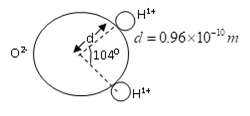Electric dipole
Question

# A molecule of water appear as shown in figure. Its dipole moment, assuming that all the electrons in the molecule rotate symmetrically about the oxygen nucleus is nearly (Take cos 520 = 0.616).

Moderate
Solution

##$P=2P\mathrm{cos}{52}^{\circ }=2\left(e\right)\left(d\right)\mathrm{cos}{52}^{\circ }$$=2×{1.0}^{-29}$

Get Instant Solutions